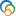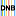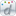## Library of Congress

### Authorities & Vocabularies

The Library of Congress > Linked Data Service > LC Subject Headings (LCSH)

# Arithmetical algebraic geometry

• ### Variants

• Algebraic geometry, Arithmetical
• Arithmetic algebraic geometry
• Diophantine geometry
• Geometry, Arithmetical algebraic
• Geometry, Diophantine

• ### Closely Matching Concepts from Other Schemes

•Arithmetical algebraic geometry•Arithmetische Geometrie•Géométrie algébrique arithmétique• ### Sources

• found: Work cat.: Current trends in arithmetical algebraic geometry, c1987.
• found: Math. subj. classif.(under Number theory: 11Gxx, Arithmetic algebraic geometry (Diophantine geometry))
• found: LC database, March 31, 1987:in titles (arithmetical algebraic geometry; diophantine geometry)
• found: Arithmetic algebraic geometry, 1991.
• notfound: ASTI;Encyc. dict. math.;CompuMath cit. index;TEST;Hennepin;James math. dict.
• ### LC Classification

• QA242.5-QA242.6
• ### Change Notes

• 1987-04-02: new
• 2000-02-28: revised

# Suggest terminology

The LC Linked Data Service welcomes any suggestions you might have about terminology used for a given heading or concept.

Would you like to suggest a change to this heading?

Please provide your name, email, and your suggestion so that we can begin assessing any terminology changes.

Fields denoted with an asterisk (*) are required.